Checkout JEE MAINS 2022 Question Paper Analysis : Checkout JEE MAINS 2022 Question Paper Analysis :

# Height And Distance Problems

## Introduction To Height And Distance

The analysis of the relationship between the sides and angles of a triangle is trigonometry. The study of height and distance can be done using trigonometry. Trigonometry has many applications, from the field of architecture to engineering to astronomy. It can be used to measure distance and angles by assuming an imaginary line connecting the points. In this article we learn different trigonometry height and distance problems with solutions.

### What is Height and Distance in Trigonometry?

The measurement of an object facing vertically is the height. Distance is defined as the measurement of an object from a point in a horizontal direction. If an imaginary line is drawn from the observation point to the top edge of the object, a triangle is formed by the vertical, horizontal and imaginary line.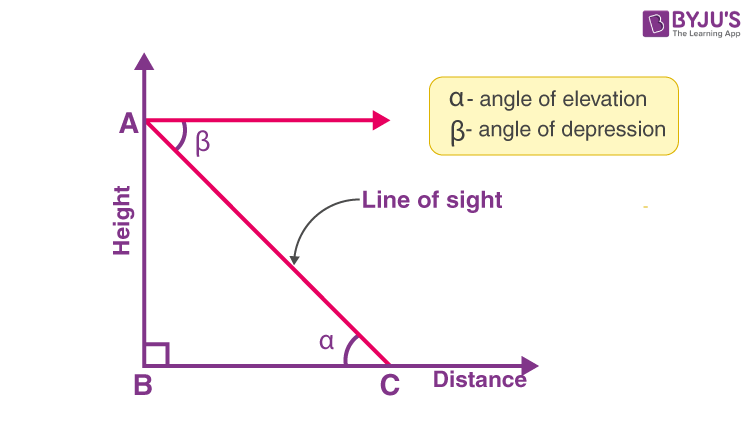From the figure, the point of observation is C. AB denotes the object’s height. BC gives the distance between the object and the observer. The line of sight is given by AC. Angles alpha and beta represent the angle of elevation and depression respectively. If any of the two quantities are provided [a side or an angle], the remaining can be found using them. The law of alternate angles states that the magnitude of the angle of elevation and angle of depression are equal in magnitude. tan α = height / distance

Method of solving a problem of height and distance

• Represent the given data of the angles and distances using a figure.
• If the problem that is to be solved is a right-angled triangle with an angle and a side known, trigonometrical ratios can be used to find the remaining angles and sides.
• The formula is the sine rule given by,
$$\begin{array}{l}\frac{a}{sin\ A} = \frac{b}{sin\ B} = \frac{c}{sin\ C}\end{array}$$
• Cosine formula i.e., cos A = [b2+ c2 − a2 ] / 2bc
• The length of an appropriate side from the 2 triangles having a side common can be obtained by equating the two values.

## Solved Problems on Height And Distance

Example 1: A vertical pole consists of two parts, the lower part being one-third of the whole. At a point in the horizontal plane through the base of the pole and distance 20 meters from it, the upper part of the pole subtends an angle whose tangent is 1/2. What are the possible heights of the pole?

Solution: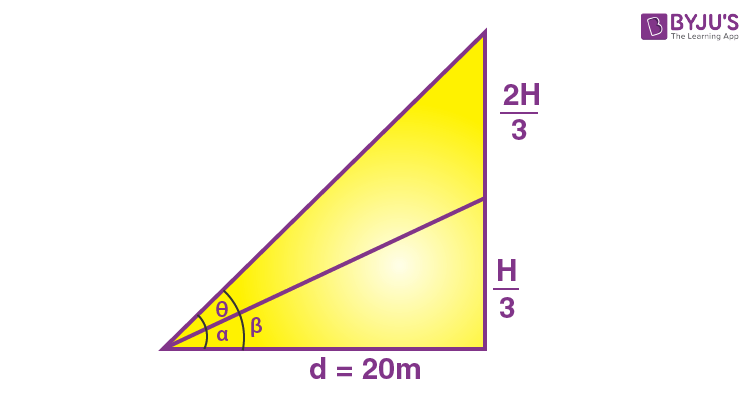tan α = H/60

tan θ = 1/2

tan β = H/20

tan (β – α) = tan θ

tan (β − α) = 1 / 2

(tan β  – tan α)/(1 + tan β tan α) = 1/2

⇒  [(H/20) – (H/60)]/(1 + H2/1200) = 1/2

⇒ (2H/60)/(1200 + H2)/1200 = 1/2

⇒ 40H/(1200 + H2) = 1/2

⇒ 80H = 1200 + H2

⇒ H2 − 80H + 1200 = 0

⇒ (H – 20) (H – 60) = 0

⇒H = 20 or 60m

Example 2: From a 60-meter high tower, the angles of depression of the top and bottom of a house are α and β respectively. If the height of the house is [60 sin (β − α)] / x, then find x.

Solution: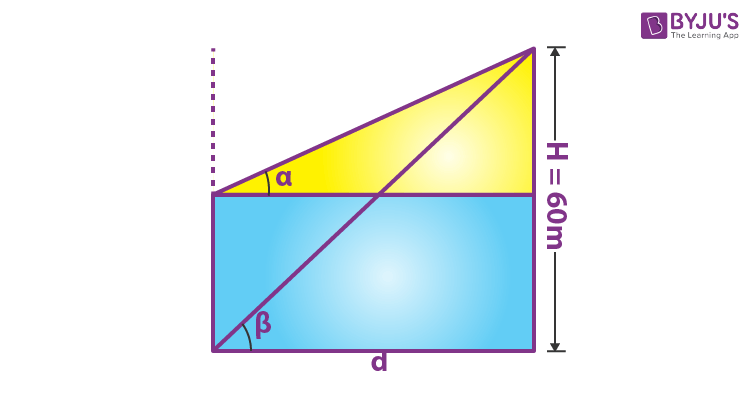H = d tan β

H − h = d tan α

⇒ (60 − h)/60 = tan α / tan β

⇒ 1 – h/60 =  tan α / tan β

⇒ h/60 = 1 – tan α / tan β

⇒ h/60 = (tan β – tan α)/tan β

⇒ h/60 = (cos α sin β – cos β sin α)/cos α sin β

⇒ h/60 = sin (β – α)/cos α sin β

Given h = 60 sin (β − α)] / x

S0 60 sin (β − α)] / 60 x = sin (β – α)/cos α sin β

⇒ x = cos α sin β

Example 3: An aeroplane flying horizontally 1 km above the ground is observed at an elevation of 60o and after 10 seconds the elevation is observed to be 30o. Find the uniform speed of the aeroplane in km/h.

Solution: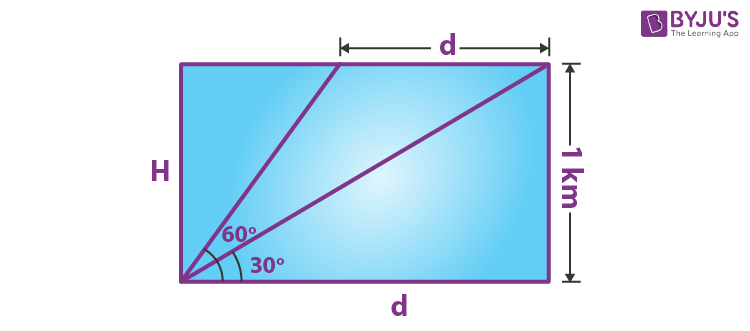d =H cot 30o −H cot 60o;

Time taken = 10 seconds = 1/360 hrs

Speed = ([cot 30o − cot 60o] / (1/360)

= (2/√3)/(1/360)

= 240√3

Example 4: At a distance 2h from the foot of a tower of height h, the tower and a pole at the top of the tower subtend equal angles. What would be the height of the pole?

Solution: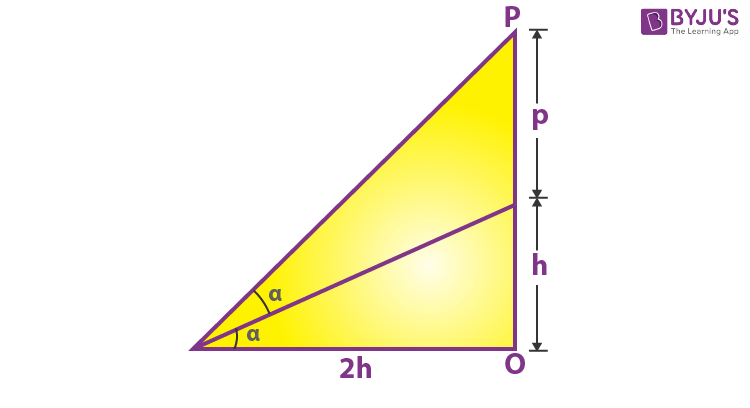tan α = 1 / 2 and PO = p+h

tan 2α = 2 tan α / 1 − tan2 α

⇒ [p + h] / [2h] = 1 / [1 − 1 / 4]

⇒ p + h / 2h = 4 / 3

⇒ p = 5h / 3

Example 5: Some portion of a 20 meters long tree is broken by the wind and the top struck the ground at an angle of 30o. The height of the point where the tree is broken is __________.

Solution: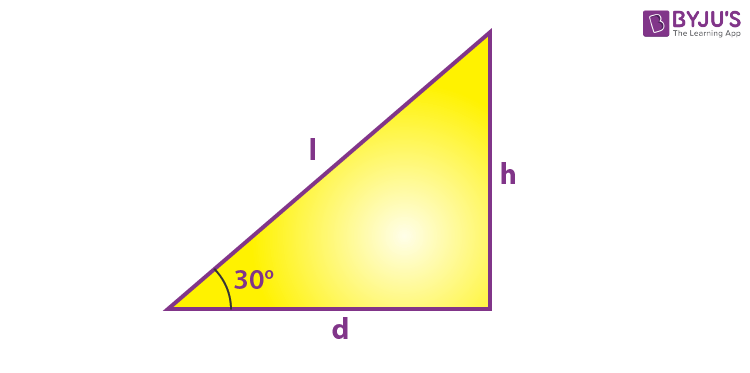Height of tree = 20 = l + h,

l = 20-h

sin 30 = h/(20-h)

1/2 = h/(20 – h)

20 – h = 2h

20 = 3h

so h = 20/3 m

Example 6: A tower of height b subtends an angle at a point O on the level of the foot of the tower and at a distance a from the foot of the tower. If a pole mounted on the tower also subtends an equal angle at O, the height of the pole is.

Solution: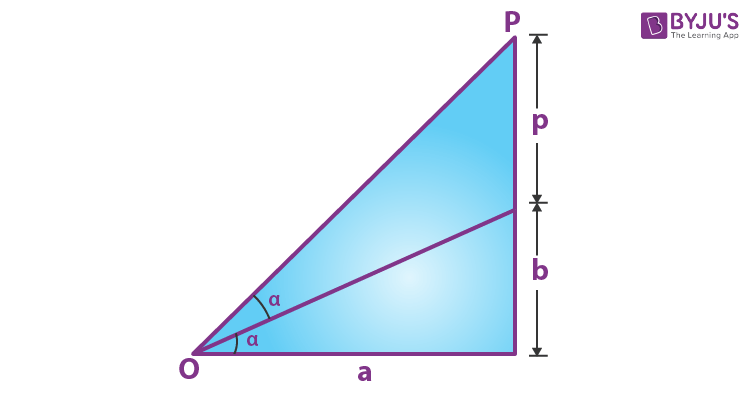Let b be the height of tower and p be the height of the pole

tan α = b / a

tan 2α = (p + b)/a

2 tan α/(1 – tanα) = (p + b)/a

⇒ 2ba / a2 − b2 = [p + b] / [a]

⇒ [2ba2 − a2b + b3] / [a2 − b2] = p

⇒ p = [b (a2 + b2)] / [(a2 − b2)]

Example 7: An observer on the top of a tree, finds the angle of depression of a car moving towards the tree to be 30 degrees. After 3 minutes this angle becomes 60 degrees. After how much more time, the car will reach the tree.

A) 4 min.

B) 4.5 min.

C) 1.5 min.

D) 2 min.

Solution: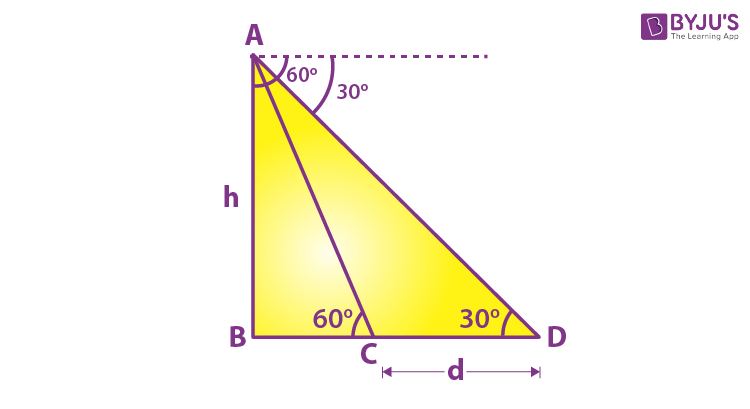In triangle ABD, BD = h cot 300

In triangle ABC, BC = h cot 600

CD = BD – BC

$$\begin{array}{l}d=h\cot {{30}^{o}}-h\cot {{60}^{o}}\text{ and time }=3 \text \ min.\end{array}$$

Therefore, Speed

$$\begin{array}{l}= \frac{h(\cot {{30}^{o}}-\cot {{60}^{o}})}{3}\end{array}$$
per minute

It will travel distance BC in

$$\begin{array}{l}\frac{h\cot {{60}^{o}}\times 3}{h(\cot {{30}^{o}}-\cot {{60}^{o}})}\end{array}$$

= 1.5 min

Example 8: A house of height 100 metres subtends a right angle at the window of an opposite house. If the height of the window be 64 metres, then the distance between the two houses is

A) 48 m

B) 36 m

C) 54 m

D) 72 m

Solution: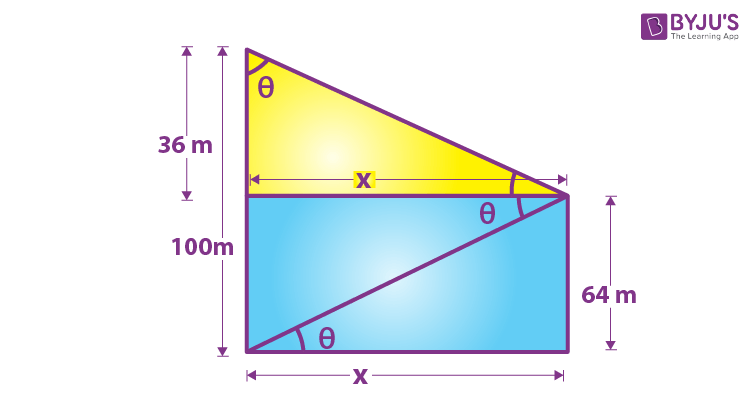tan θ = 64/x…(i)

tan θ = x/36…(ii)

equating (i) and (ii)

64/x = x/36

x2 = 2304

so x = 48 m

The distance between the houses is 48 m.

Example 9:  From a point h metre above a lake the angle of elevation of a cloud is θ and the angle of depression of its reflection is ϕ. The height of the cloud is

Solution: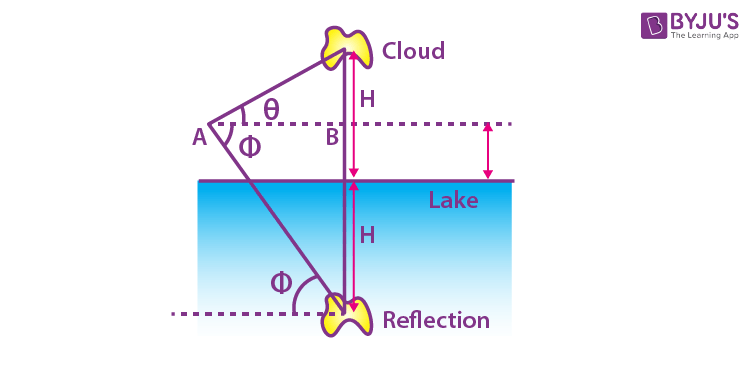Let H be the height of the cloud from the surface of the lake.

(H-h)/AB = tan θ

(H+h)/AB = tan ϕ

(H-h)/(H+h) = tan θ/tan ϕ

(H-h+H+h)/(H-h-H-h) = (tan θ + tan ϕ)/(tan θ – tan ϕ)

2H/-2h = (tan θ + tan ϕ)/(tan θ – tan ϕ)

H = -h(tan θ + tan ϕ)/(tan θ – tan ϕ)

= h(tan θ + tan ϕ)/(tan ϕ – tan θ)

Example 10: At a point on the ground the angle of elevation of a tower is such that its cotangent is 3/5. On walking 32 metres towards the tower the cotangent of the angle of elevation is 2/5. The height of the tower is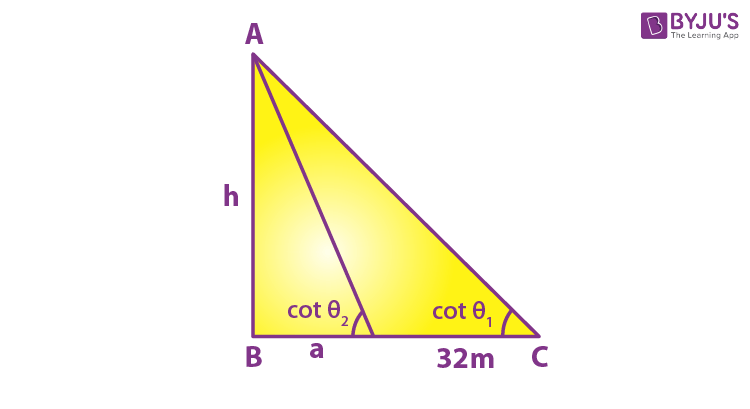A) 160 m

B) 120 m

C) 64 m

D) None of these

Solution:

cot θ1 = 3/5

cot θ2 = 2/5

cot θ1 = (32 + a)/h

cot θ2 = a/h

so a = 2h/5

3/5 = (32 + 2h/5)/h

=> 3h = 32×5 + 2h

=> h = 160m

Example 11: A person standing on the bank of a river observes that the angle of elevation of the top of a building on the opposite bank is 450. When he moves 20m away from the bank, he finds the angle of elevation to be 300. Find the height of the building.

Solution:

Let PR represent the height of the building and PS represents the river.

QS = 20 m

Angle of elevation at S is 450 and at Q is 300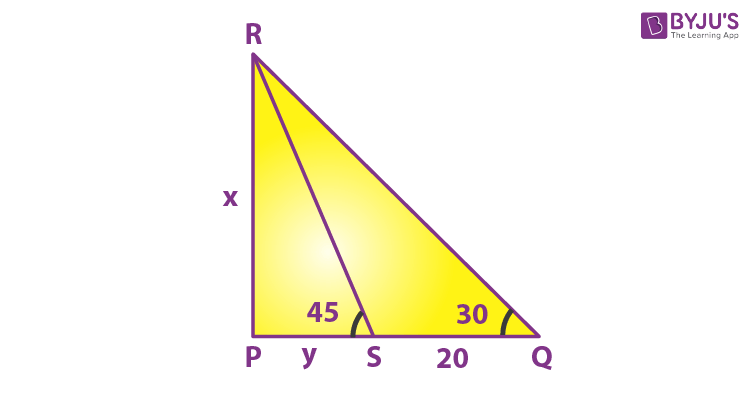In Δ PRS, tan 45 = x/y

⇒1 = x/y

⇒ x = y

In  Δ PQR, tan 30 = x/(20+y)

⇒  1/√3 = x/(20+x)  [ since x = y]

⇒ 20+x = √3x

x(√3-1) = 20

x = 20/(√3-1)

Multiply numerator and denominator by (√3+1)

⇒x = 20(√3+1)/(√3-1)(√3+1)

⇒x =  20(√3+1)/(3-1)

⇒x =  20(√3+1)/2

⇒x = 10(√3+1) m

Hence the height of the building is  10(√3+1) m.

### What do you mean by angle of elevation?

The angle of elevation is an angle formed between the horizontal line and the line of sight, when an observer looks upwards.

### What do you mean by angle of depression?

If the object is below the level of the observer, then the angle formed between the horizontal line and the observer’s line of sight is called the angle of depression.

### Give the importance of Angle of Elevation and Angle of Depression.

Angle of elevation and Angle of Depression are used to solve distance and height problems in trigonometry when a line of sight is involved.

Test your Knowledge on Height and distance